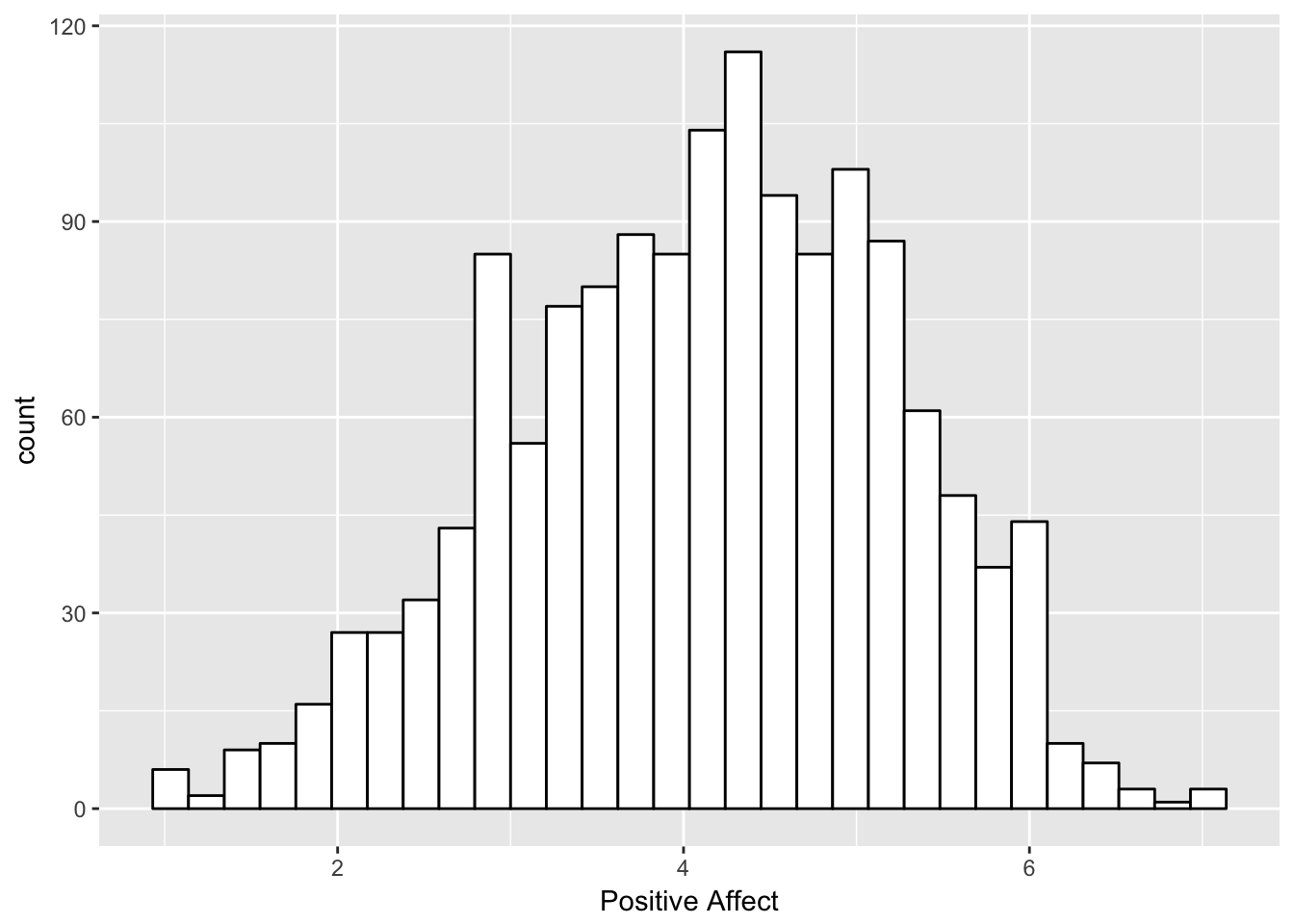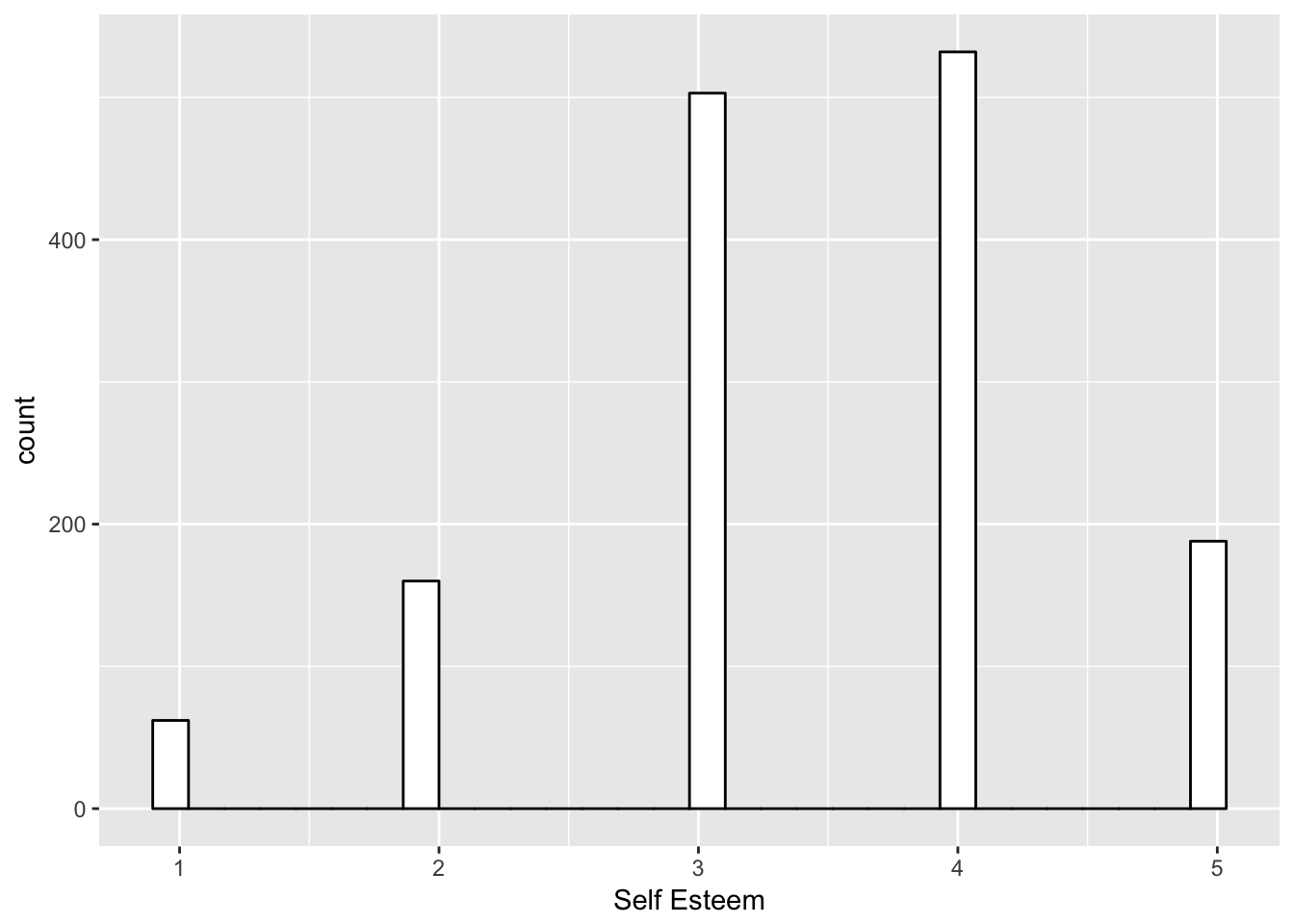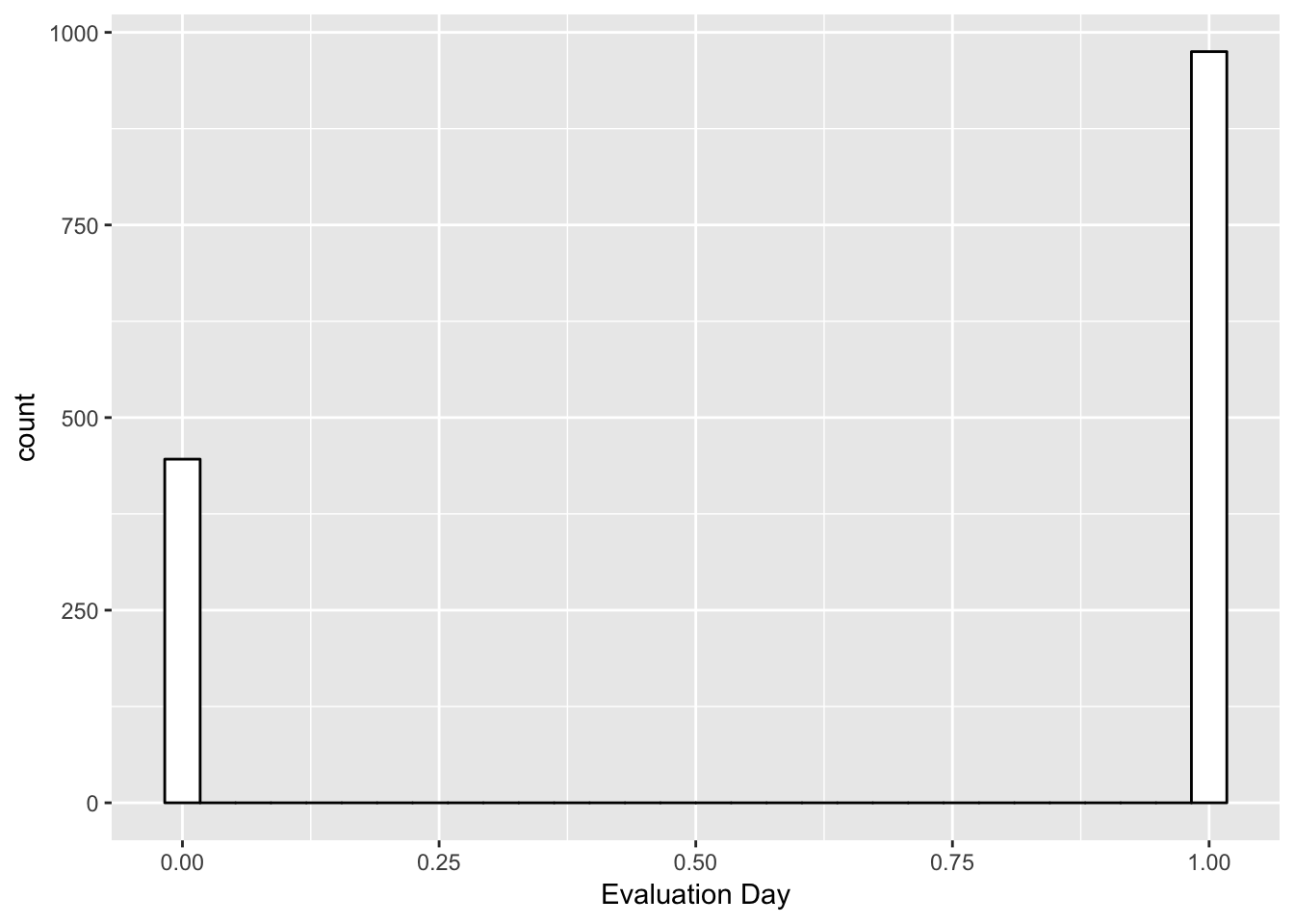## Overview

This tutorial walks through calculation of a few intraindividual variability metrics. Specifically, this tutorial demonstrates calculation of intraindividual means, standard deviations, counts, proportions, and entropy. These metrics are useful for articulating a variety of dynamic characteristics from experience sampling data - a sometimes useful set of interindividual differences constructs

## Outline

In this session we cover …

A. Looking at distributions and within-person time-series (continuous, categorical, binary)
B. Calculating within-person summaries (imean, isd, etc.)
C. Calculating using a formula - (ientropy)

``````library(psych)
library(ggplot2)
library(data.table)
library(plyr)
library(entropy)``````

#### Prelim - Reading in Repeated Measures Data

Note that we are working from a long file. For your own data, there may be some steps to get to this point.

``````#Setting the working directory
setwd("~/Desktop/Fall_2017")  #Person 1 Computer
#setwd("~/Desktop/Fall_2017")  #Person 2 Computer

#set filepath for data file
filepath <- "https://quantdev.ssri.psu.edu/sites/qdev/files/AMIBbrief_raw_daily1.csv"
#read in the .csv file using the url() function

#Little bit of clean-up
var.names.daily <- tolower(colnames(daily))
colnames(daily)<-var.names.daily``````

Everything we do today uses a long file

A reminder …
1. How many unique persons are there in these data?

``````#Make a vector of the unique ids
idlist <- unique(daily\$id)
#length of the id vector
length(idlist)``````
``##  190``

Ok so, N = 190.

1. How many observations are there in these data?
``````#Make a vector of the unique ids
daylist <- unique(daily\$day)
#length of the id vector
length(daylist)``````
``##  8``

Ok so, T = 8.

## A: Looking at distributions and within-person time-series (continuous, categorical, binary)

Lets look at some descriptions of today’s variables.

``````#getting a list of the variable names
names(daily)``````
``````##   "id"      "day"     "date"    "slphrs"  "weath"   "lteq"    "pss"
##   "se"      "swls"    "evalday" "posaff"  "negaff"  "temp"    "hum"
##  "wind"    "bar"     "prec"``````
``````#sample descriptives
describe(daily\$posaff)``````
``````##    vars    n mean  sd median trimmed  mad min max range  skew kurtosis
## X1    1 1441 4.12 1.1    4.2    4.15 1.19   1   7     6 -0.25    -0.33
##      se
## X1 0.03``````
``describe(daily\$se)``
``````##    vars    n mean   sd median trimmed  mad min max range  skew kurtosis
## X1    1 1445 3.43 0.99      3    3.47 1.48   1   5     4 -0.41    -0.11
##      se
## X1 0.03``````
``describe(daily\$evalday)``
``````##    vars    n mean   sd median trimmed mad min max range skew kurtosis   se
## X1    1 1421 0.69 0.46      1    0.73   0   0   1     1 -0.8    -1.36 0.01``````
``````#histograms
ggplot(data=daily, aes(x=posaff)) +
geom_histogram(fill="white", color="black") +
labs(x = "Positive Affect")``````
``## `stat_bin()` using `bins = 30`. Pick better value with `binwidth`.``
``## Warning: Removed 17 rows containing non-finite values (stat_bin).````````ggplot(data=daily, aes(x=se)) +
geom_histogram(fill="white", color="black") +
labs(x = "Self Esteem")``````
``## `stat_bin()` using `bins = 30`. Pick better value with `binwidth`.``
``## Warning: Removed 13 rows containing non-finite values (stat_bin).````````ggplot(data=daily, aes(x=evalday)) +
geom_histogram(fill="white", color="black") +
labs(x = "Evaluation Day")``````
``## `stat_bin()` using `bins = 30`. Pick better value with `binwidth`.``
``## Warning: Removed 37 rows containing non-finite values (stat_bin).``The histograms display the various measurement scales of these variables (interval, ordinal, binary).

And lets look at the longitudinal (“spaghetti”) plots …
1. Positive Affect

``````#ggplot version .. see also http://ggplot.yhathq.com/docs/index.html
ggplot(data = daily[which(daily\$id <=105),], aes(x = day, y = posaff, group = id, color=factor(id))) +
geom_point() +
geom_line(data=daily[which(daily\$id <= 105 & daily\$posaff !="NA"),]) +
xlab("Day") +
ylab("Positive Affect") + #ylim(1,7) +
scale_x_continuous(breaks=seq(0,7,by=1)) ``````